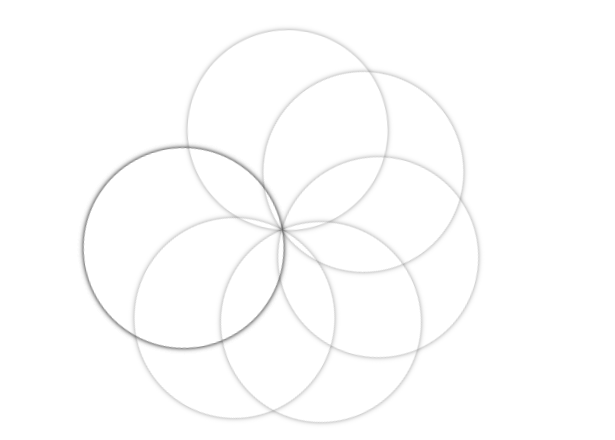# How to create rotating disc effect using CSS ?

• Last Updated : 05 Jun, 2020

The Rotating Disc Effect also known as Overlapping Disc Effect is a type of illusion effect that can be used for various purposes on a website. It can be used in anything from a loader sticker to creating an illusion for the user. It is called overlapped disc because there are many overlapped discs which are rotating around a single point.

Approach: The approach is to create all the discs first and then use keyframes and n-th child properties to rotate them.

HTML Code: In this section, we have created a un-ordered list(ul) with list-items(li) inside it. The number of list-items is equal to the number of discs.

 ` ` `<``html` `lang``=``"en"``> ` `  ``<``head``> ` `    ``<``meta` `charset``=``"UTF-8"` `/> ` `    ``<``meta` `name``=``"viewport"` `content``= ` `        ``"width=device-width, initial-scale=1.0"` `/> ` `    ``<``title``>Rotating Disc Effect ` ` `` ` `  ``<``body``> ` `    ``<``ul``> ` `      ``<``li``> ` `      ``<``li``> ` `      ``<``li``> ` `      ``<``li``> ` `      ``<``li``> ` `      ``<``li``> ` `      ``<``li``> ` `      ``<``li``> ` `    `` ` `  `` ` ` `

CSS Code: For CSS, follow the given steps.

• First thing is to create disc by using the border-radius property. Set the border-radius to 50% to create a perfect circle.
• Now, use animation with an identifier to be used later with keyframes. We have used animate as our identifier.
• Now, use keyframes to rotate the disc for each frames. Here we have used 0deg for first frames and 359deg for second frame.
• Now, use n-th child property to apply a delay of 1st to each disc. It helps to rotate each disc with some delay that is responsible for the illusion kind of effect.

Tip: You can apply different opacity colors to each disc to make it look more appealing.

 ` `

Complete Code: It is the combination of the above two sections of code.

 ` ` `<``html` `lang``=``"en"``> ` `  ``<``head``> ` `    ``<``meta` `charset``=``"UTF-8"` `/> ` `    ``<``meta` `name``=``"viewport"` `content``= ` `        ``"width=device-width, initial-scale=1.0"` `/> ` `    ``<``title``>Rotating Disc Effect ` `    ``<``style``> ` `      ``ul { ` `        ``margin: 0; ` `        ``padding: 0; ` `        ``position: absolute; ` `        ``left: 40%; ` `        ``top: 20%; ` `      ``} ` ` `  `      ``ul li { ` `        ``list-style: none; ` `        ``width: 200px; ` `        ``height: 200px; ` `        ``position: absolute; ` `        ``top: 0; ` `        ``border-radius: 50%; ` `        ``box-shadow: 0 0 5px rgba(0, 0, 0, 0.5); ` `        ``animation: animate 7s linear infinite; ` `        ``transform-origin: bottom center; ` `      ``} ` ` `  `      ``@keyframes animate { ` `        ``0% { ` `          ``transform: rotate(0deg); ` `        ``} ` `        ``100% { ` `          ``transform: rotate(359deg); ` `        ``} ` `      ``} ` ` `  `      ``ul li:nth-child(1) { ` `        ``animation-delay: 0s; ` `      ``} ` `      ``ul li:nth-child(2) { ` `        ``animation-delay: 1s; ` `      ``} ` `      ``ul li:nth-child(3) { ` `        ``animation-delay: 2s; ` `      ``} ` `      ``ul li:nth-child(4) { ` `        ``animation-delay: 3s; ` `      ``} ` `      ``ul li:nth-child(5) { ` `        ``animation-delay: 4s; ` `      ``} ` `      ``ul li:nth-child(6) { ` `        ``animation-delay: 5s; ` `      ``} ` `      ``ul li:nth-child(7) { ` `        ``animation-delay: 6s; ` `      ``} ` `      ``ul li:nth-child(7) { ` `        ``animation-delay: 7s; ` `      ``} ` `    `` ` `  `` ` `  ``<``body``> ` `    ``<``ul``> ` `      ``<``li``> ` `      ``<``li``> ` `      ``<``li``> ` `      ``<``li``> ` `      ``<``li``> ` `      ``<``li``> ` `      ``<``li``> ` `      ``<``li``> ` `    `` ` `  `` ` ` `

Output:My Personal Notes arrow_drop_up
Recommended Articles
Page :